##### International Journal of
###### eISSN: 2576-4454# HydrologyResearch Article Volume 4 Issue 2

# Evaluation of temperature-based solar radiation models and their impact on penman-monteith reference evapotranspiration in a semiarid climate

#### Koffi Djaman,1 function clickButton(){ var name=document.getElementById('name').value; var descr=document.getElementById('descr').value; var unCopyslNo=document.getElementById('unCopyslNo').value; document.getElementById("mydiv").style.display = "none"; $.ajax({ type:"post", url:"https://medcraveonline.com/captchaCode/server_action", data: { 'name' :name, 'descr' :descr, 'unCopyslNo': unCopyslNo }, cache:false, success: function (html) { //alert('Data Send');$('#msg').html(html); } }); return false; } Verify Captcha Regret for the inconvenience: we are taking measures to prevent fraudulent form submissions by extractors and page crawlers. Please type the correct Captcha word to see email ID.function refreshPage(){ $("#mydiv").load(location.href + " #mydiv"); }$(document).ready(function () { //Disable cut copy paste $('#msg').bind('cut copy paste', function (e) { e.preventDefault(); }); //Disable mouse right click$("#msg").on("contextmenu",function(e){ return false; }); }); .noselect { -webkit-touch-callout: none; /* iOS Safari */ -webkit-user-select: none; /* Safari */ -khtml-user-select: none; /* Konqueror HTML */ -moz-user-select: none; /* Firefox */ -ms-user-select: none; /* Internet Explorer/Edge */ user-select: none; /* Non-prefixed version, currently supported by Chrome and Opera */ cursor: none; } Lamine Diop,2 Komlan Koudahe,3 Ansoumana Bodian,2 Papa Malick Ndiaye2

1Agricultural Science Center at Farmington, Department of Plant and Environmental Sciences, New Mexico State University, P.O. Box 1018, Farmington, NM 87499, USA
2Université Gaston Berger, BP 234-Saint-Louis, Sénégal
3ADA Consulting Africa, 07 BP 14284 Lomé, Togo

Correspondence: Koffi Djaman, Agricultural Science Center at Farmington, Department of Plant and Environmental Sciences, New Mexico State University, P.O. Box 1018, Farmington, NM 87499, USA, Tel +1-505-960-7757

Received: April 18, 2020 | Published: April 30, 2020

Citation: Djaman K, Diop L, Koudahe K, et al. Evaluation of temperature-based solar radiation models and their impact on Penman-Monteith reference evapotranspiration in a semiarid climate. Int J Hydro. 2020;4(2):84-95. DOI: 10.15406/ijh.2020.04.00230

# Abstract

Keywords: solar radiation, prediction, reference evapotranspiration, semiarid climate

# Introduction

Crop evapotranspiration (ETc) is an important parameter in hydrological, environmental and agricultural studies and plays a key role in designing and managing irrigation projects and water management under irrigated and rainfed agriculture. While crop ETc is directly measured with lysimeters, Bowen ratio energy balance system and Eddy covariance systems,1921 it is also estimated by the indirect method using reference crop evapotranspiration (ETo) and crop coefficients as proposed by Jensen.2224 Evapotranspiration is one of the largest components of the hydrological cycle and is expected to increase with the warming across the Southwestern United States.24 Water resources are limited under semiarid regions similar to the Southwestern United States, the hottest and driest region in the United States with diminishing winter and spring precipitation and shifts in precipitation and reference evapotranspiration.2528 This projection is mostly challenging regarding water resources management and planning when the human population is increasing along with the demand for food and an increasing competition among water users such as agricultural producers, industries, mines, communities, environmentalists and others. Prein et al.29 indicated that the southwestern United States climate may be transitioning to a drier climate state leading to higher drought risk. To cope with the aforementioned climatic conditions and the future projection, accurate estimation of crop water use may be a priority for water management and planning under conservative agriculture. Reference evapotranspiration (ETo) is estimated by different methods from direct measurements with lysimeters and Eddy covariance system to indirect modeling using local climatic variables.4,3037 Numerous ETo equations have been developed with different parameterizations. Their performance and adaptability vary throughout the globe and the Penman-Monteith equation is shown to be the most worldwide accurate under all types of climatic conditions.34,3846 However, the number of climatic variables required by the Penman-Monteith equation is the most limiting constraint for its adoption under limited data conditions. As aforementioned, solar radiation is the most scared measured variable and the simulation of this variable could partly help overcome data limitation for the Penman-Monteith equation adoption to improve water management under changing climate. The objectives of the present study were to (1) evaluate the performance of the nineteen existing and one developed temperature based solar radiation models and (2) evaluate the impact of the predicted solar radiation estimates on the Penman-Monteith grass reference evapotranspiration as a case study in a semiarid climate of the state of New Mexico.

# Materials and methods

Study area

This study was conducted at five weather stations across New Mexico (USA): Alcade, Fabian-Garcia, Farmington, Leyendecker and Tucumcari for the period of 2009-2017. The geographical coordinates and the long term average climatic variables at each station are summarized in Table 1. Minimum temperature (Tmin), maximum temperature (Tmax), minimum relative humidity (RHmin), maximum relative humidity (RHmax), wind speed (u2), and solar radiation (Rs) were collected on the daily basis from automated weather stations installed by the New Mexico Climate Center. The time series data were check for quality control following the methodology described by Allen et al.34

 Parameters Alcalde Fabian garcia Farmington Leyendecker Tucumcari Latitude (Deg. North) 36.09 32.28 36.69 32.20 35.20 Longitude (Deg. West) -106.06 -106.77 -108.31 -106.74 -103.69 Elevation (m) 1735 1186 1720 1176 1246 Wind speed (m/s) 1.19 1.82 2.45 1.81 3.30 Tmax (°C) 21.09 26.50 20.94 26.12 24.07 Tmin (°C) 2.22 9.82 4.33 7.67 7.52 Tmean (°C) 11.65 18.16 12.63 16.89 15.79 RHmax (%) 83.86 65.21 68.63 77.34 71.78 RHmin (%) 22.88 18.60 19.70 19.09 22.29 RHmean (%) 53.37 41.90 44.17 48.21 47.03 Rs (MJ m-2) 18.62 21.23 19.84 19.63 19.80

Table 1 Weather stations with long term average climatic condition

Empirical methods to estimate daily solar radiation

Twenty empirical methods were used to estimate daily solar radiation in this research. One simple equation with any exponet (equation 21) was developped in this study to facilited solar radiation estimation with very basic tools as most of the ranchers across the study area are within the upper age class and have limited accress to the highly efficiency estimation tools. The selected models are:

Hargreaves and Samani3 and Allen47

$Rs=a*{\left(\frac{p}{101.3}\right)}^{0.5}*�{T}^{0.5}*Ro$       (1)

(2)

Bristow-Campbell5

$Rs=a*\left(1-{e}^{b{\left(�T\right)}^{0.5}}\right)*Ro$      (3)

Hargreaves and Samani4

$Rs=a*�{T}^{0.5}*Ro$      (4)

Clemence48

Rs= a  (b*Ro*T+c Tmax T+d T+e)      (5)

$Rs=a*\left(b*RoT+c*Tmax*T+d*T+e\right)$     (6)

Hunt et al.10

$Rs=a*Ro*�{T}^{0.5}+b$        (7)

Ertekin-Yaldiz49

$Rs=a+b*Ro+c*T$         (8)

Samani6

(9)

Annandale et al.50

$Rs=a*\left(1+2.7*{10}^{-5}*Z*�{T}^{0.5}\right)*Ro$    (10)

Chen et al.9 named Chen1

$Rs=Ro*\left(a*ln\left(\Delta T\right)+b\right)$   (11)

Chen et al.9 named Chen2 Hargreaves et al.51

$Rs=Ro*\left(a*�{T}^{0.5}+b\right)$    (12)

El-Sebaii et al.52

Rs=(a+b*T+c*RH)*Ro    (13)

Benghanem and Mellit.53

Rs=a*Ro*∆T0.25+b     (14)

Hassan et al.8

$Rs=Ro*\left(a+b*T\right)*�{T}^{C}$     (15)

Rao et al.54

$Rs=a*Ro*{e}^{b{\left(�T\right)}^{0.5}}$     (16)

Jahani et al.1

$Rs=Ro*\left(a+b*�T+c*{\left(�T\right)}^{2}+d*{\left(�T\right)}^{3}$      (17)

Fan et al.13

$Rs=Ro*\left(a+b*�T+c*{\left(�T\right)}^{0.25}+d*{\left(�T\right)}^{0.5}$      (18)

Nage et al.55 named Nage1

$Rs=\left(a*�{T}^{0.7}*R{o}^{1.3}+b\right)*Ro$      (19)

Nage et al.55 named Nage2

$Rs=\left(a+b*�{T}^{0.5}+c*\mathrm{ln}\left(�T\right)\right)*Ro$     (20)

Richardson et al.56

(21)

Djaman et al.

$Rs=\left(a+b*�T\right)*Ro$     (22)

where Ro=the daily extraterrestrial solar radiation (MJm-2) determinded following the procedure described by Allen et al.34; 𝞓T=(Tmax-Tmin); T=(Tmax+Tmin)/2; T, 𝞓T, Tmax and Tmin in oC; RH=mean relative humidity (%); Z=station altitude (m); a, b, c, d and e are dimenssionless empirical constants to be determined during the calibration process.

Penman-monteith reference evapotranspiration

Daily grass-reference evapotranspiration was computed using the standardized ASCE form of the Penman–Monteith equation38 (Equation 22):

(23)

where, ETo is reference evapotranspiration (mm/day), Δ is the slope of saturation vapor pressure versus air temperature curve (kPaoC-1), Rn=net radiation at the crop surface (MJ m-2 d-1), G=soil heat flux density at the soil surface (MJ m-2 d-1), Tmax and Tmin are maximum and minimum air temperature (0C), T=mean daily air temperature at 1.5-2.5m height (oC), u2=mean daily wind speed at 2m height (m s-1), es=the saturation vapor pressure (kPa), ea=the actual vapor pressure (kPa), es-ea=saturation vapor pressure deficit (kPa), γ=psychrometric constant (kPa oC-1),. Rs=solar radiation (MJ m-2 d-1). All parameters necessary for computing ETo were computed according to the procedure developed in FAO-56 by Allen et al.34

Calibration of the daily solar irradiation models

Evaluation of the impact of solar radiation simulated by empirical models on the Penman-Monteith reference evapotranspiration estimates

The measured and predicted daily solar radiation were interchanged in combination with all other required weather variables (Tmax, Tmin, RHmax, RHmin and U2) to estimate daily ETo under both conditions. The Penman-Monteith ETo (ETo-PM-meas) estimates using the measured daily solar radiation was compared to the one using the predicted daily solar radiation by the temperature based solar radiation models (ETo-PM-pred) using simple linear regression with the intercept forced to be zero. The evaluation criteria used for solar radiation models evaluation are also used for the evaluation of the impact of the solar radiation models on the Penman-Monteith reference evapotranspiration estimates. In addition, Student T-test was used to compare the ETo-PM-meas and ETo-PM-pred across all models and weather stations to determine any significant difference between the original ETo estimates and the predicted ETo values using predicted solar radiation values at 95% level of confidence.

# Results and discussion

Calibration and evaluation of the solar radiation models

The selected solar radiation models showed different performance and accuracy at each of the five weather stations under this study (Figures 1‒3). The best fitting constants that optimized the daily solar radiation estimation at each weather station and for each of the selected models are summarized in Table 2. The boxplots of the performance criteria are presented in Figure 1. The Kling-Gupta efficiency (KGE) varied with model and locations and ranged from 0.69 to 0.91 (Figure 2). The best model at all five weather stations combined with the greatest accuracy was the Hassan’s model obtaining an average medium KGE value of 0.90 followed by Fan et al., Bist Camp, Samani and Cichar and the models. The lowest KGE values were obtained for the Benghamen, EsSebaii, Ert Yal and Clemence models (Figure 1). Clemence showed the lowest medium KGE value of 0.79. All models performed better at Farmington followed by Tucumcari, Fabian Garcia and Alcalde (Figure 2). Considering average KGE value, Hassan’s model was the best performing model (KGE value=0.89) and Benghamen and Clemence are the least performing models with average KGE value of 0.80.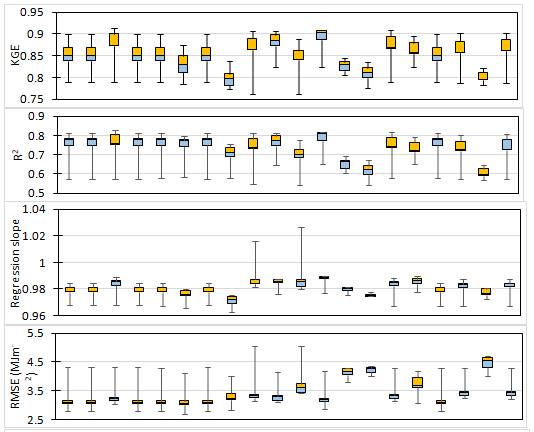Figure 1 Boxplots of the statistics evaluation criteria for solar radiation models at five weather stations (pooled) in New Mexico.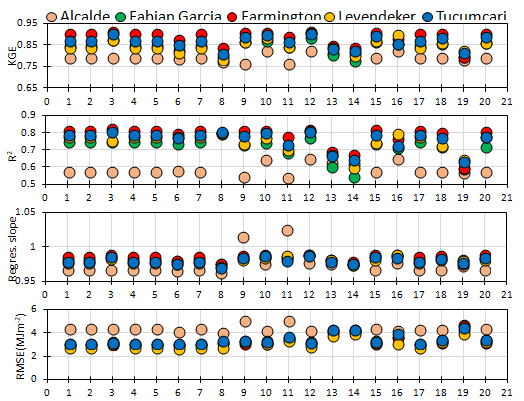Figure 2 KGE, R2, regression slope, root mean squared error (RMSE), mean bias error (MBE), mean absolute error (MAE) and Nash-Sutcliffe model efficiency coefficient (NSE) between the measured and the predicted daily solar radiation using different solar radiation models at Alcalde. Fabian Garcia, Farmington, Leyendecker, and Tucumcari. The order of the solar radiation models 1, 2, 3…, 20 is the same as in Figure 1.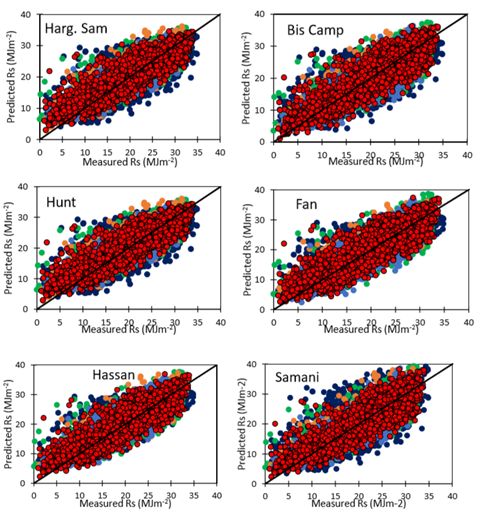Figure 3 Regression plotting between the measured and the predicted daily solar radiation by the best eight solar radiation models at Alcalde. Fabian Garcia, Farmington, Leyendecker, and Tucumcari.

 Solar radiation models Coeff. 1 2 3 4 5 Average Solar radiation models Coeff. 1 2 3 4 5 Average Hargreaves and Samani (1982) a 0.15985 0.18135 0.17575 0.16014 0.17637 0.17069 Rao et al. (2017) a 0.22648 0.17053 0.19466 0.14497 0.14785 0.17690 Bristow-Campbell (1984) a 0.82118 1.23935 1.03183 1.28326 1.25769 1.12666 b 0.23193 0.34040 0.29181 0.34302 0.36613 0.31466 b 0.03628 0.02876 0.03163 0.02516 0.02771 0.02991 Johani et al. (2017) a 0.40000 0.43213 0.44606 0.40000 0.38743 0.41312 c 2.17650 1.7256 1.89798 1.50981 1.66258 1.79449 b 0.00014 0.00014 0.00014 0.00014 0.00014 0.00014 Hargreaves and Samani (1985) a 0.14417 0.16907 0.15865 0.14938 0.16384 0.15702 c 0.00066 0.00085 0.00066 0.00066 0.00092 0.00075 Hunt et al. (1998) a 0.14417 0.16907 0.15865 0.14938 0.16384 0.15702 d 0 0 0 0 0 0 b 0 0 0 0 0 0 Fan et al. (2018) a 0.37133 0 0 0 0 0.07427 Ertekin-Yaldiz (1999) a 0 0 0 0 0 0 b 0.00799 0.01538 0.00004 0.01359 0.02006 0.01141 b 0.50343 0.48641 0.53594 0.42944 0.47701 0.48645 c 0 0 0 0 0.12785 0.02557 c 0.30923 0.32963 0.27483 0.37626 0.3513 0.32825 d 0.00000 0.08203 0.15072 0.05612 0.00018 0.05781 Samani (2000) a 0.11559 0.13913 0.15193 0.11559 0.127 0.12985 e 0.25630 0.16629 0.07692 0.26751 0.13563 0.18053 b 0.00177 0.00177 8e-05 0.00177 0.00214 0.00151 Nage et al. (2018) 1 a 0.00019 0.00024 0.00021 0.00024 0.00024 0.00022 c 0 0 0 0 0 0 b 0.48551 0.51747 0.49682 0.45949 0.50041 0.49194 Annandale et al. (2002) a 0.13772 0.16383 0.15161 0.14478 0.15851 0.15129 Nage et al. (2018) 2 a 0 0 0 0 0 0 Chen et al. (2004) 1 a 0.13907 0.16907 0.15865 0.14938 0.16384 0.15600 b 0.11963 0.16907 0.15865 0.14938 0.16384 0.15212 b 0.02209 0 0 0 0 0.00442 c 0.03651 0 0 0 0 0.00730 Chen et al. (2004) 2 a 0 0 0 0 0 0 Richardson et al. (2018) a 0.15314 0.10283 0.12145 0.07889 0.09021 0.10931 b 0.21455 0.24573 0.23176 0.22096 0.23895 0.23039 b 0.47953 0.67739 0.59416 0.71851 0.71144 0.63621 El-Sebaii et al. (2009) a 0.52989 0.55801 0.55265 0.50131 0.53993 0.53636 Clemence a 0.37093 0.43187 0.44511 0.42100 0.35052 0.40389 b 0.00683 0.00608 0.00647 0.00716 0.00681 0.00667 b 0.10016 0.08149 0.06678 0.08435 0.07407 0.08137 c 0 0 0 0 0 0 c 1.12916 1.68926 1.18619 1.58008 1.22095 1.36113 Benghanem and Mellit. (2014) a 0.30076 0.33958 0.32166 0.30877 0.33034 0.32022 d 0.09515 0.08493 0.06842 0.08593 0.05850 0.07859 b 0 0 0 0 0 0 e 15.70956 0 13.90342 0 14.99549 8.92170 Hassan et al. (2016) a 0.29538 0.14109 0.13856 0.13149 0.12809 0.16692 Djaman a 0.30346 0.19989 0.25003 0.15982 0.16040 0.21472 b 0.00309 0.00091 0.00024 0.00138 0.00058 0.00124 b 0.01678 0.02923 0.02348 0.02582 0.03014 0.02509 c 0.20984 0.52149 0.53844 0.48282 0.55926 0.46237

Table 2 Constants of the selected solar radiation models derived at the five weather stations from the fitting calibration using Solver in Excel
1, Alcalde; 2, Fabian Garcia; 3, Farmington; 4, Leyendecker; 5, Tucumcari;

The coefficient of determination of the regression between the measured and the predicted daily solar radiation estimates was dependent on the model. The boxplots of R2 showed that most of the models obtained moderately high values except for the El-Sebaii, Ert Yal and Clemence models with R2 values lower than 0.70. However, when most models showed boxplot high median R2 value, the minimum R2 values were quite low between 0.54 and 0.58. All models showed the highest R2 values at Farmington weather station and the lowest R2 values at Alcalde weather station (Figure 2). The highest and the lowest values of R2 were showed by Bist Camp model and Johani model, respectively (Figure 1).

High regression slope between the measured and the predicted daily solar radiation values were obtained and varied from 0.96 and 1.03 (Figure 2). The boxplots showed that the regression slope values are lower than unity for most of the models with the boxes below the y=1 line while Samani and Johani showed maximum value greater than unity, indicating solar radiation overestimation at Alcalde (Figure 2). Hassan’s model was still the best performing model and the Berghamen’s model showed the lowest regression slope. All models showed the lowest regression slope at Alcalde except Samani and Johani models.

Root mean square error (RMSE) of the predicted daily solar radiation estimates varied within a wide range between 2.67 and 5.71MJm-2 as shown in Figure 2. RMSEvalues were concentrated between 3 and 3.5MJm-2 for most of the models except for Johani, El Sebaii, Ert-Yal, Nage1 and Clemence models which showed higher RMSE values (Figure 1). Overall, models showed the lowest RMSE values at Leyendecker while they showed the highest RMSE values at Alcalde and the intermediate RMSE values were obtained at Farmington and Tucumcari. The Samani and Johani models showed the highest RMSE at Alcalde and the lowest RMSE value was shown by Chen2 at Leyendecker (Figure 2).

The mean absolute error (MAE) ranged from 2.36 to 3.87MJm-2 (Figure 2). The boxplots showed low MAE with most of the boxes within the range of 2.2–2.7 (Figure 1). Johani, El-Sebaii, Ert YAl, Nage1 and Clemence models showed great MAE while all models showed the maximum MAE greater than 3MJm-2 at Alcalde (Figure 1). Similar to the RMSE, the highest MAE values were obtained at Alcalde and the lowest MAE were registered at Leyendecker (Figure 2). Overall, the model Chen2 showed the lowest MAE value of 2.40MJm-2 and the highest MAE value of 3.26MJm-2 was obtained by Clemence model. The mean bias error (MBE) were quite low and ranged between -0.01 and 1.07MJm-2. Only Samani and Johani models showed MBE values of 0.85 and 1.08MJm-2 at Alcalde, respectively, and Johani showed MBE value of 0.18MJm-2 at Leyendecker as the highest MBE values (Figure 2). The Nash-Sutcliffe model efficiency coefficient (NSE) varied from 0.49 to 0.83 while most of the NSE boxplots are within the range of 0.75-0.83. El-Sebaii, Ert Yal and Clemence models have the boxes below 0.7 and the Samani and Johani models showed the lowest values of NSE (Figure 2). The lowest values of the NSE recorded at Alcalde for all models and very clustered values were observed at Fabien Garcia, Farmington, Leyendecker and Tucumcari (Figure 2).

Impact of the predicted solar radiation on the Penman-Monteith reference evapotranspiration

Daily grass reference evapotranspiration was estimated by the Penman-Monteith equation using the measured solar radiation and the predicted solar radiation by the selected solar radiation models. The impact of the solar radiation prediction accuracy on the performance of the Penman-Monteith equation was drawn and the results showed that solar radiation prediction accuracy had a little effect on the reference evapotranspiration estimates. The coefficient of determination of the simple linear regression line between the ETo-PM-meas and the ETo-PM-pred varied with locations and solar radiation model used. It ranged from 0.94 to 0.99 and the highest R2 value were observed at Farmington and Tucumcari for all solar radiation models. The lowest R2 values were registered at Alcalde (Figure 4).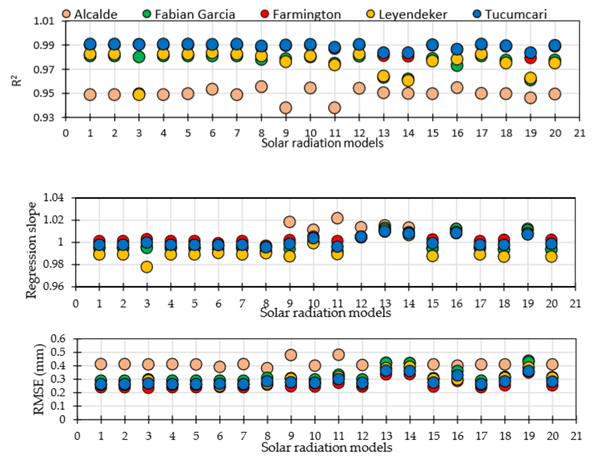Figure 4 R2, regression slope, root mean squared error (RMSE), mean bias error (MBE), and mean absolute error (MAE) and Nash-Sutcliffe model efficiency coefficient (NSE) between the original and the predicted daily reference evapotranspiration using predicted daily solar radiation with the different models at Alcalde, Fabian Garcia, Farmington, Leyendecker, and Tucumcari. The order of the solar radiation models 1, 2, 3…, 20 is the same as in Figure 1.

The regression slope varied from 0.978 to 1.022 showing the relatively high correlation between the two sources of ETo estimates. Slight overestimation of ETo was mostly observed at Farmington with a regression slope greater than unity however, the highest ETo overestimation was obtained at Alcalde when daily solar radiation was predicted by the Samani, Fan, El-Sabaii, and Johani models. Daily ETo was systematically overestimated at all five stations when the solar radiation was predicted by the Hassan, El Sebaii, Ert Yal, Nage1 and Clemence models (Figure 4). Overall, the lowest regression slope was obtained at Leyendecker and the maximum ETo overestimation and underestimation equal to 2.2% seems to be reasonable.

Low RMSE of ETo estimates were obtained and varied from 0.24 to 0.48mm/day with the highest RMSE observed at the Alcalde (Figure 4). Similarly, very low MAE and MBE values were observed and ranged from 0.15 to 0.31mm/day (Figure 4) and from -0.03 to 0.09mm/day (Figure 4), respectively. The highest MAE values were obtained at Alcalde and the lowest values were obtained at Farmington (Figure 4) while the lowest MBE values were obtained at Leyendecker and the highest MBE value were not systematically obtained at only one station. Solar radiation predicted by Samani, Fan et al, Hassan, El-Sebaii, ERt Yal, Nage1 and Clemence models are sources of the high MBE of the predicted ETo values. The overall performance of the solar radiation models showed non-significant impact on the ETo estimates and the Nash-Sutcliffe model efficiency coefficient (NSE) values were quite high and varied from 0.93 to 0.99 with the lowest values of NSE observed at Alcalde and the highest NSE values observed at Farmington and Tucumcari across all solar radiation models (Figure 4). NSE values averaged 0.95, 0.98. 0.99, 0.98 and 0.99 at Alcalde, Fabian Garcia, Farmington, Leyendecker and Tucumcari, respectively.

The Student T-test revealed non-significant differences between the original and the predicted ETo values across all five weather stations using any of the 20solar radiation models. Consequently, the different solar radiation models have non-significant impact on estimating daily grass reference evapotranspiration. The good fitness of the predicted ETo versus the observed ETo estimates is shown in Figure 5 presenting the impact of the best eight solar radiation models on ETo estimates. These results are in agreement with Tabari et al.62 and Bandyopadhyay et al.61 who reported that the solar radiation prediction model choice had a little impact on the reference evapotranspiration estimates. Tabari et al.62 reported no noticeable improvement in ETo estimates when daily solar radiation data was predicted by the Hargreaves-Samani, Allen, Samani, Annandale, Chen and Ertekin-Yaldiz models with Penman-Monteith ETo deviation range between from -2.16 to 1.40mm between the original and the calibrated solar radiation.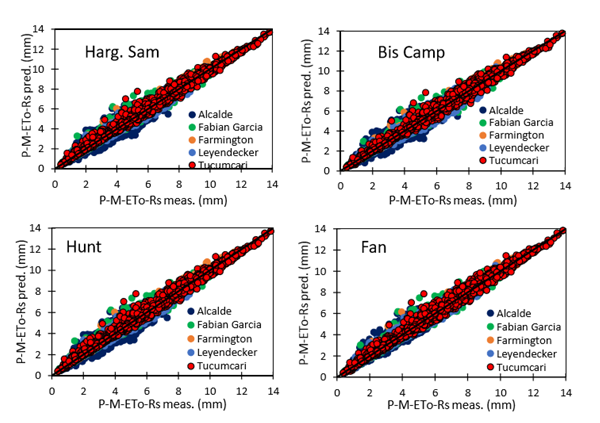Figure 5 Regression plotting between the original Penman-Monteith ETo with the measured solar radiation and the predicted Penman-Monteith ETo with the predicted solar radiation by the best eight solar radiation models at Alcalde, Fabian Garcia, Farmington, Leyendecker, and Tucumcari.

# Conclusion

Performance and accuracy of twenty temperature based solar radiation models were evaluated at five weather stations in New Mexico for the global period of 2009-2017 using the optimization procedure Solver in Excel which maximized the Kling-Gupta Efficiency. The results showed that the Hargreaves and Samani3 improved by Allen,47 Bristow-Campbell,5 Hunt et al.,10 Fan et al.,13 Hassan et al.,8 Samani,6 Nage55 2, and Richardson56 models were the most accurate and the best performing ones across all research sites with the highest Kling-Gupta Efficiency, the root mean squared error (RMSE), mean absolute error (MAE), mean bias error (MBE), and the Nash-Sutcliffe model efficiency coefficient. The evaluation of the impact of the predicted solar radiation on the Penman-Monteith reference evapotranspiration showed that the predicted solar radiation had non-significant effect of the daily reference evapotranspiration with regression slopes varying from 0.978 to 1.022, RMSE from 0.24 to 0.48mm/day, MAE from 0.15 to 0.31mm/day and MBE from -0.03 to 0.09mm/day. These best performing solar radiation models could be considered as the alternatives for estimating daily solar radiation across New Mexico when solar radiation is missing and for predicting daily reference evapotranspiration across the study area.

# Acknowledgments

We completed this work with the support of New Mexico State University (NMSU) and the Agricultural Science Center at Farmington and all the staff.

# Conflicts of interest

The authors declare no conflict of interest.

None.

# References©2020 Djaman, et al. This is an open access article distributed under the terms of the, which permits unrestricted use, distribution, and build upon your work non-commercially.